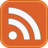# James Percival

## My Github Pages material

Last updated : 30th August 2018

# My blog - by most recent

Full index or by tag
• Running an X11 connection with docker

It’s conceivable that you might want to run an X11 based application inside a docker container. If the host system is a mac with XQuartz installed, then this StackOverflow post contains the kernel of one method to do so. Note that getting this running may require modifying your XQuartz defaults by running the command

defaults write org.macosforge.xquartz.X11 enable_iglx -bool true


in the terminal.

• A basic Dockerfile

Lets write ourselves a generic Dockerfile using build-args to update an image for apt based distros

• Using Docker on a mac

There are now several different ways to run Docker containers on an Apple Mac. First, there’s an app for that Docker for Mac. Second, there’s command line options, which spin up a VirtualBox virtual machine running boot2docker and run the actual image/container from inside there.

• Note to self - disabling whitespace in liquid

It appears that when generating static websites using liquid, the default syntax,

• Adding tags to a Github Pages blog

Today I got round to adding tag support to this blog. The code to process the tags page looks like the following:

Adding navigation buttons to a layout is really easy. First the .css to generate the buttons and arrows:

• Installing Delft3d on an Apple Mac

Delft3d is “a world leading 3D modeling suite to investigate hydrodynamics, sediment transport and morphology and water quality for fluvial, estuarine and coastal environments.”, available for multiple operating systems. However it can be surprisingly difficult to get it running on OSX.

• The Exner equation - Part one

Lets derive the Exner equation from first principles. Consider two surfaces, $$z=\eta(x,y)$$, denoting the boundary of a sediment bed and $$z=h(x,y)$$, denoting the free surface of the water column, as well as a curve in the x-y plane, $$\mathcal{C}(x,y)$$, bounding a cylinder, $$\Omega\times\mathbb{R}$$, in space. The total amount of sediment contained in this cylinder in this viewpoint can be written as $S = \int_{\Omega} \left[\int_{-\infty}^{\eta} (1-\phi) dz+ \int_\eta^{\eta+\Delta} c_b^*\,dz +\int_{\eta+\Delta}^h c\,dz\right]dy\,dx.$ where $$\phi(x,y,z)$$ is the bed porosity, $$c_b^*$$ is the bedload sediment and $$c$$ is the suspended sediment. Taking a time derivative, and applying the Leibnitz integral rule gives $\frac{d S}{dt} = \int_\Omega \left( (1-\phi)\frac{\partial \eta}{\partial} dy\,dx + \frac{d}{dt} \left[ \int_\eta^{\eta+\Delta} c_b^*\,dz +\int_{\eta+\Delta}^h c\,dz\right] \right) dy\,dx.$ Similarly we can consider the flux of material through the boundary, $$\mathcal{C}$$, $\frac{d S}{dt} = \oint_\mathcal{C} \left[\int_\eta^{\eta+\Delta} \left( c_b^* \mathbf{u}_b -\kappa_b \nabla c_b^*\right)\cdot \mathbf{n} \,dz + \int_{\eta+\Delta}^{h} \left( c \mathbf{u} -\kappa\nabla c \right) \cdot\mathbf{n}\,dz\right]d\ell.$ Applying the divergence theorem and making the twin ansatzes that $\Delta$ does not change with time and that changes in $$c_b$$ can be neglected, we obtain the relation $(1-\phi)\frac{\partial \eta}{\partial t} + \nabla \cdot \mathbf{q}_b + \frac{d}{dt}\int_{\eta+\Delta}^h c\,dz +\nabla\cdot \int_{\eta+\Delta}^h \left( c\mathbf{u} -\kappa \nabla c\right)\,dz=0,$ where $$\mathbf{q}_b$$ is the total flux in the bedload layer, $\mathbf{q}_b = \int_\eta^{\eta+\Delta} \left( c_b^* \mathbf{u}_b -\kappa_b \nabla c_b^* \right) \cdot \mathbf{n} \,dz.$ If we consider just the sediment in the water column, and assume that no sediment is lost through the free surface we see $\frac{d}{dt}\int_{\eta+\Delta}^h c\,dz +\nabla\cdot \int_{\eta+\Delta}^h \left( c\mathbf{u} -\kappa \nabla c \right) = F, dz$ where $$F$$ is the inward flux of material at the bottom of the free water column.

Note that we have deliberately not written $$F$$ as $$\left(c\mathbf{u}-\kappa\nabla\right)\cdot\mathbf{\hat{z}}$$, since it might arise from a different modelling process than the turbulence modelling being applied inside the free water column. Substituting this into the total sediment conservation law gives the final version of the Exner equation, $(1-\phi)\frac{\partial \eta}{\partial t} + \nabla \cdot \mathbf{q}_b = -F.$

Effectively the volume of sediment lost or gained by the bed must be balanced by a transfer of material to or from the suspended sediment, while the bedload tranport exists only to move, not hold material. It remains to define the bedload flux, $$\mathbf{q}_b$$ and the flux into the water column, as well as better describing the physics within the water column itself.

• Example of scour modelling through hr mesh adaptivity
• Mesh, Velocity and vorticity for flow past a cylinder at around Re = 25.
Full index or by tag